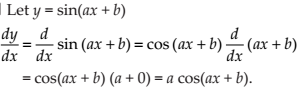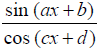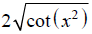Sunday, August 9, 2020
Home > CBSE Class 12 > NCERT Solutions for Class 12 Maths Continuity and Differentiability Exercise 5.2

# NCERT Solutions for Class 12 Maths Continuity and Differentiability Exercise 5.2# NCERT Solutions for Class 12 Maths Continuity and Differentiability

Hi Students, Welcome to Amans Maths Blogs (AMB). In this post, you will get the NCERT Solutions for Class 12 Maths Continuity and Differentiability Exercise 5.2. This NCERT Solutions can be downloaded in PDF file. The downloading link is given at last.

CBSE Class 12th is an important school class in your life as you take some serious decision about your career. And out of all subjects, Maths is an important and core subjects. So CBSE NCERT Solutions for Class 12th Maths is major role in your exam preparation as it has detailed chapter wise solutions for all exercise.

NCERT Solutions for Class 12 Maths are not only the solutions of Maths exercise but it builds your foundation of other important subjects. Getting knowledge of depth concept of CBSE Class 12th Maths like Algebra, Calculus, Trigonometry, Coordinate Geometry help you to understand the concept of Physics and Physical Chemistry.

As we know that all the schools affiliated from CBSE follow the NCERT books for all subjects. You can check the CBSE NCERT Syllabus. Thus, NCERT Solutions helps the students to solve the exercise questions as given in NCERT Books.

## NCERT Solutions for Class 12 Maths Continuity and Differentiability Exercise 5.2

Differentiate the functions with respect to x in Exercises 1 to 8.

NCERT Solutions for Class 12 Maths Continuity and Differentiability Exercise 5.2: Ques No 1.

sin (x2 + 5)

NCERT Solutions:NCERT Solutions for Class 12 Maths Continuity and Differentiability Exercise 5.2: Ques No 2.

cos (sin x)

NCERT Solutions:NCERT Solutions for Class 12 Maths Continuity and Differentiability Exercise 5.2: Ques No 3.

sin (ax + b)

NCERT Solutions:NCERT Solutions for Class 12 Maths Continuity and Differentiability Exercise 5.2: Ques No 4.

sec (tan (√x))

NCERT Solutions:NCERT Solutions for Class 12 Maths Continuity and Differentiability Exercise 5.2: Ques No 5.NCERT Solutions:NCERT Solutions for Class 12 Maths Continuity and Differentiability Exercise 5.2: Ques No 6.

cos x3 . sin2 (x5)

NCERT Solutions:NCERT Solutions for Class 12 Maths Continuity and Differentiability Exercise 5.2: Ques No 7.NCERT Solutions:NCERT Solutions for Class 12 Maths Continuity and Differentiability Exercise 5.2: Ques No 8.

cos(√x)

NCERT Solutions:NCERT Solutions for Class 12 Maths Continuity and Differentiability Exercise 5.2: Ques No 9.

Prove that the function f given by f (x) = | x – 1 |, x ∈ R is not differentiable at x = 1.

NCERT Solutions:NCERT Solutions for Class 12 Maths Continuity and Differentiability Exercise 5.2: Ques No 10.

Prove that the greatest integer function defined by f (x) = [x], 0 < x < 3 is not differentiable at x = 1 and x = 2.

NCERT Solutions:error: Content is protected !!